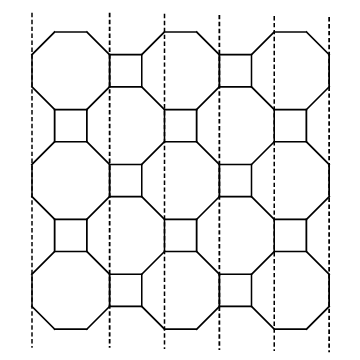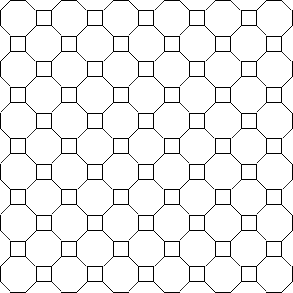The maze book for programmers!

Algorithms, circle mazes, hex grids, masking, weaving, braiding, 3D and 4D grids, spheres, and more!

# The Buckblog

## Upsilon Mazes

28 November 2015 — A walk-through of how to implement a grid and maze on an octagon/square tiling — 9-minute read

Let’s talk about grids formed by combining octogons and squares in what is called a truncated square tiling (also called a truncated quadrille). This kind of tiling gives you a nifty kaleidoscope effect:Mazes made on this style of grid are called upsilon mazes for some reason, but at least it’s easier to say than octagon-and-square mazes. We’ll stick with “upsilon”, and name the grid that as well: upsilon mazes, and upsilon grids.

Upsilon grids have very well-defined horizontal and vertical rows and columns, unlike hexagon and triangle grids. But where most other grids have a single shape for every cell, upsilon grids need to represent both squares and octogons. This complicates things a little bit, but honestly, it’s not that bad.

Let’s implement one of these grids together, so you can see what I mean. We’ll start at the bottom, implementing the cells. (For those of you with my book, feel free to implement this using the framework I introduced there. For this blog post, though, I’m going to assume people are doing the work from scratch.)

## Implementing the Cells

As mentioned, upsilon grids are composed of two different shapes: octagons and squares. We’ll create a base `Cell` class (which provides just basic functionality, like forging links between two adjacent cells), and then we’ll subclass that for our `OctagonCell` and `SquareCell` concrete subclasses.

The abstract `Cell` class looks like this:

The subclasses, then, just implement accessors for querying each of a cell’s neighbors, as well as a method that returns all of the cell’s neighbors at once:

So far, so good! Let’s see how these play together in a grid, next.

## Configuring the Grid

Our `UpsilonGrid` class will have this basic structure (we’ll flesh out the methods as we go):

Looking at the `initialize` method, you’ll see we’re calling `#_setup_grid` to create the grid itself, and we’ll use `#_configure_cells` to describe which cells are adjacent to each other.

Let’s take these one a time.

If you look long enough at an illustration of an upsilon grid, you can see that the octagons and squares alternate.One row will start with an octagon, and the next with a square. (It’s actually the same alternating pattern exhibited by the orientation of the cells on a triangle grid, from page 122 of my book.) There’s a simple rule we can follow to determine the shape for each cell: `OctagonCell` when the row and column sum to an even number, and `SquareCell` otherwise.

We can use that rule in `#_setup_grid` to populate our cell collection, like this:

Just so! Our `@grid` variable is now a two-dimensional array of alternating octagons and squares. Next, we just need to iterate over each of those cells and tell them who their neighbors are. It’s not hard at all (just a little verbose):

Nothing to it, but brace yourself. This is just the calm before the storm. Next we’re going to dig into what it takes to actually display this thing.

## Measuring the Grid

First, we’ll walk through the measurements needed to specify an octagon by its center point, and then show an implementation of `#to_png` that will draw the grid onto a canvas for us.

The key to deriving the points of the octagon relative to its center point is simply to split the octagon into triangles. Give it a try, if you’re so inclined, but note that because regular octagons don’t decompose to equilateral triangles, the math isn’t as neat as it is for, say, hexagons.

For brevity sake, here’s how it all breaks down on an octagon.Let’s have each side of the octagon be of length s, and let c be the center point of the octagon. It’s clear from this that the lines drawn through c split those corresponding sides in half, so A must be s/2. And if we were to go through that little derivation I glossed over, we’d see that B must be equal to s/√2.

That’s all we need to know, then, to compute the x- and y-coordinates of each of the octagon’s vertices! If we take far to mean “furthest from the center”, and near to mean “nearest to the center”, then we can compute those coordinates like this:

Using those coordinates, then, we can specify the location of each of the octagon’s vertices. For instance, the vertex near the 10 o’clock position on the perimeter would be at `(x_west_far, y_north_near)`.

All that’s left, then, is to be able to calculate the size of our canvas. (The `#to_png` method has to allocate a canvas to draw on, so we’ll need to be able to derive it’s dimensions.) It’s not too hard; it just requires that we slice up the grid a particular way. Consider this:Given a 5x5 upsilon grid (three octagons and two squares in each dimension), we count one whole octagon width first, followed by one B-sized slice and a square-sized slice (size s) for each column. The height works out similarly. Our canvas dimensions, then, can be computed as follows:

Whew! Alright, let’s implement this.

## Displaying the Grid

We have enough now to take a stab at the `#to_png` implementation. We’re actually going to be adding two new methods in addition to the `#to_png` method: one for drawing the octagons and one for drawing the squares.

First, though, here’s the `#to_png` method. It just computes the constants we derived above, and allocates the canvas. Then it iterates over each cell and calls the appropriate drawing method.

The next method, `#_draw_octagon_cell`, is tasked with drawing the relevant walls of a single `OctagonCell`.

Lastly, we have the `#_draw_square_cell`. It’s much shorter than the octagonal version, but works on the same principle: compute the corners, and draw the walls.

Now, let’s see if we did it right!

## Making Upsilon Mazes

Let’s start by just drawing the grid itself. If we’ve coded everything up right, the following ought to do the trick:

Odd-numbered dimensions tend to look better with upsilon grids, giving them a nice symmetry where each row and column starts and ends with the same shape, but feel free to experiment with different dimensions. Our 11x11 upsilon grid should look something like this:Success!

Of course, we can’t stop there. We’re so close to having an actual upsilon maze! With a just a few more lines of code, we can apply the Recursive Backtracking algorithm, to build a maze on this thing. Try this:Looks good to me!

Try a few other maze algorithms with it and see what different effects you get. You may want to give it a try with Binary Tree and Sidewinder here, as well. The octagons and squares tile in a vaguely rectangular fashion, which means you can make both work simply by treating the octagons as squares and ignoring the diagonals. With a bit of thought, though, it’s even possible to use some of the diagonals with these algorithms.

Good luck!

P.S. If you want to play with the code I developed in this article, and you don’t want to go through the hassle of pasting it all together, I’ve put it all in a gist. Grab it here!